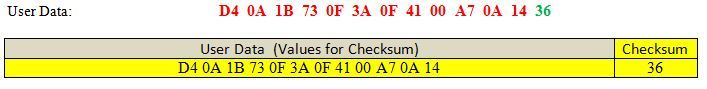# How to calculate 8-bit Checksum (2’s complement)?

• Greetings,

I am receiving data via Bluetooth, and I want to calculate 8-bit Checksum (2’s complement) for incoming data (Hex format).
what is the simplest approach for this calculation?

I am using the following code for incoming data interpretation:

``````    while (socket->bytesAvailable())
{

char hex[hexString.size()];
strcpy(hex, hexString);
QByteArray data=QByteArray::fromHex(hex);
QDataStream stream(data);
stream.setByteOrder(QDataStream::BigEndian);

uint8_t bn, nb, esc, s, cs;
uint16_t d1,d2,d3,d4;

stream>>bn>>nb>>esc>>s;
stream>>d1>>d2>>d3>>d4;
stream>>cs;
}
``````

Looking forward to your kind responses.

• @ahsan737
You'll have to be more specific about just what you mean. "Hex format" is not relevant, as per your code you'll convert any strings into the actual numbers and do the math on that. 8-bit checksum is often just `sum() % 255` or `(uint8_t)sum()`, perhaps you want a `~` in front of it for your "2’s complement". See maybe https://stackoverflow.com/questions/31151032/writing-an-8-bit-checksum-in-c or https://stackoverflow.com/questions/27505240/computing-a-8-bit-checksum-in-c.

``````            char hex[hexString.size()];
strcpy(hex, hexString);
QByteArray data=QByteArray::fromHex(hex);
``````

is simply a waste of time & space, you can just `QByteArray::fromHex(hexString);`. Worse that that, you are actually copying one more character (the terminating `\0`) from `hexString` than the size you have allocated for stack variable `hex`, so you're lucky if this does not corrupt your stack....

As a side note your, code is assuming that `socket->readAll()` inside `socket->bytesAvailable()` will read all bytes, sufficient for all your expected variables (and no more than that). This is dangerous, `readAll()` only returns however many happen to be there at the instant you call `bytesAvailable()`, which may not yet be all sent (or could even be more than for one structure, depending on your protocol). You should buffer your input till you have enough for your structure, you could look at `QDataStream::startTransaction()`.

• @JonB
Thank you so much for pointing out mistakes.
for checksum: I have to calculate checksum for given data and make comparison with the checksum reference value.• @ahsan737
You haven't said how that `36` is calculated from the input bytes. I already suggested to you

is often just `sum() % 255` or `(uint8_t)sum()`, perhaps you want a `~` in front of it for your "2’s complement".

You can do it from here, I'm not going to figure how you come up with your checksum number or do the C++ for you.

• Hi, should be something like this:

``````QByteArray receivedBytes = socket->readAll();

char c = 0;
c += b;

c = 256 - c;
qDebug() << QString::number(c,16);
``````

(I got 36 when i tested with

`````` QByteArray receivedBytes = QByteArray::fromHex("D4 0A 1B 73 0F 3A 0F 41 00 A7 0A 14")
``````

• @JonB Thanks, got your point. And I have already mentioned checksum type twice (question title, and description).

• c = 256 - c;
qDebug() << QString::number(c,16);

Slight correction: This generates a number in range `1--256`. Isn't the checksum supposed to be a byte `0--255` (`uint8_t cs`)? Yet you say you did get `36` on the data, so perhaps not, but surprises me....

• @hskoglund
thank you for such a detailed response. It holds true for other data strings that I have tested.

• @JonB No worries, a char has only 8 bits so the value 256 will be truncated to 0, i.e. the range will still be 0..255.
Basically you're translating from signed arithmetic to unsigned d:o using 2's-complement.

• @hskoglund
Yes I know that (bits, signed, overflow wrapping), I thought the result would be wrong. E.g. if there is, say, just 1 byte with value `0`, then `256 - c` will generate `256 == char(0) == 0x00` for the checksum. I expected that to need to be `255 - c` => `255 == char(255) == 0xFF` for the 2's complement? So I don't get it. But since your answer matches what the OP expects, there you are....

EDIT OK, I looked up https://en.wikipedia.org/wiki/Two's_complement

The two's complement is calculated by inverting the digits and adding one

I didn't know about the "adding one"! :) I thought it was just `~number`, got it now!

• @JonB response is correct.

From the code I posted earlier, you can add:

``````// checksum = 36h 54d
uint8_t c=0;
for(uint8_t v : data) c+=v;
c-=cs;  // remove the checksum value
c=~c;   // 1's complement
c++;    // 2's complement

if(c != cs)
{
qDebug()<<"checksum error";
}
``````

• @mpergand
thank you very much.